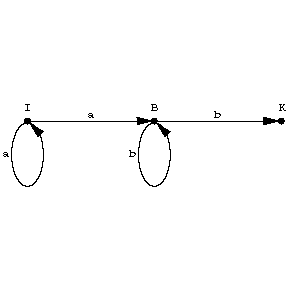# Grammar, regular

finite-state grammar, automatic grammar, left- (right-) linear grammar

A context-free grammar (cf. Grammar, context-free) each rule of which has the form $A \rightarrow aB$ or $A \rightarrow a$, where $A$ and $B$ are non-terminal symbols and $a$ is one of the terminal symbols. (Rules of the form $A \rightarrow \Lambda$, where $\Lambda$ is the empty string, are also sometimes allowed; the class of languages generated is then extended only at the expense of the languages obtained by adding the string $\Lambda$ to the previous languages.) For each regular grammar it is possible to construct a finite automaton (cf. Automaton, finite) equivalent to it. The class of languages generated by regular grammars (regular or automatic languages) coincides with the class of regular sets if rules with an empty right-hand side are allowed. The regular languages form a proper subclass of the class of linear languages (cf. Grammar, linear). Thus, the linear language $\{ {a ^ {n} b ^ {n} } : {n = 1, 2 ,\dots } \}$ is not regular. The class of regular languages is closed under union, intersection, concatenation, substitution, and truncated iteration (and, in the presence of rules with an empty right-hand side, also with respect to iteration). The intersection of a context-free language with a regular language is again a context-free language.

A regular grammar is usually illustrated by a transition diagram — an oriented multi-graph whose vertices are non-terminal symbols, and a vertex $A$ is connected to a vertex $B$ by means of an arc, marked with the (terminal) symbol $a$, if $A \rightarrow aB$ is a rule of the grammar; in addition, the transition diagram contains one more terminal vertex, connected with a non-terminal symbol $A$ by means of an arc marked by the symbol $a$ if $A \rightarrow a$ is a rule of the regular grammar. (If there are rules of the form $A \rightarrow \Lambda$, each symbol $A$ governed by this rule is also considered to be a terminal vertex.)Figure: g044840a

A string in the basic vocabulary is derivable in the grammar from a non-terminal symbol $A$ if and only if it is "written down" in some path in the diagram going from $A$ to the terminal vertex. The figure represents a transition diagram of a regular grammar with the rules $I \rightarrow aI$, $I \rightarrow aB$, $B \rightarrow bB$, $B \rightarrow b$( $I$ is the initial symbol, $K$ is the terminal vertex), which generates the language $\{ {a ^ {n} b ^ {m} } : {n, m = 1, 2 ,\dots } \}$.

How to Cite This Entry:
Grammar, regular. Encyclopedia of Mathematics. URL: http://encyclopediaofmath.org/index.php?title=Grammar,_regular&oldid=47119
This article was adapted from an original article by A.V. Gladkii (originator), which appeared in Encyclopedia of Mathematics - ISBN 1402006098. See original article